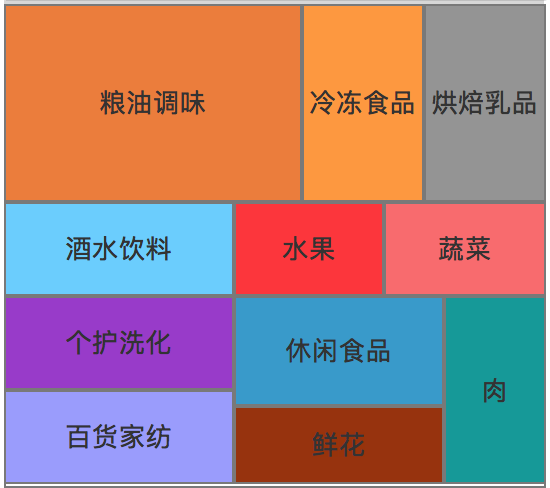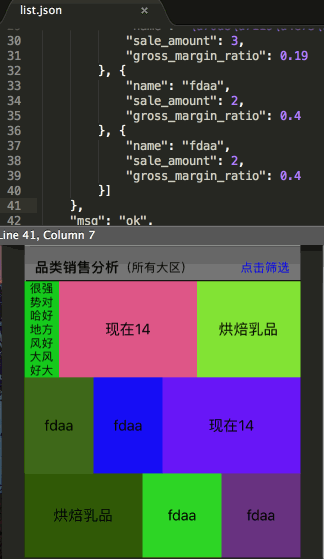# iOS实现比例拼图的方法示例

更新时间：2018年07月11日 11:30:48   作者：无夜之星辰我要评论```{
"result": true,
"data": {
"category_sale": [ {
"name": "我是你的哥",
"sale_amount": 1,
"gross_margin_ratio": 0.22
}, {
"name": "不是亲哥哥",
"sale_amount": 4,
"gross_margin_ratio": 0
}, {
"name": "呵呵哒",
"sale_amount": 3,
"gross_margin_ratio": 0.19
}, {
"name": "因缺思厅",
"sale_amount": 2,
"gross_margin_ratio": 0.4
}]
},
"msg": "ok",
"code": 200,
"executed": "0.0320830345"
}``````- (void)setModel:(CQCategoryModel *)model {
_model = model;

CGFloat totalSaleAmount = 0;
for (CQCategoryItemModel *itemModel in _model.category_sale) {
totalSaleAmount += itemModel.sale_amount;
}

for (CQCategoryItemModel *itemModel in _model.category_sale) {
if (totalSaleAmount == 0) {
// 特殊处理只有一个item，并且SaleAmount还是0的情况
itemModel.ratio = 1;
} else {
itemModel.ratio = itemModel.sale_amount/totalSaleAmount;
}
}

// 计算列数
NSInteger listCount = 0;
for (int i = 0; i < _model.category_sale.count; i++) {
if (i * i < _model.category_sale.count && (i+1) * (i+1) >= _model.category_sale.count) {
listCount = i+1;
break;
}
}

// 计算行数
NSInteger rowCount = ceil(_model.category_sale.count / (CGFloat)listCount);

// 这个方阵是listCount*rowCount的矩阵（最后一排可能不足listCount）
// 同一排的cell高度相同
for (int i = 0; i < rowCount; i++) { // 行
CGFloat rowArea = 0; // 行面积
for (int j = 0; j < listCount; j++) { // 列
if (i*listCount+j>=_model.category_sale.count) {
break;
}
CQCategoryItemModel *itemModel = _model.category_sale[i*listCount+j];
itemModel.size = itemModel.ratio * (self.collectionView.width*self.collectionView.width);
rowArea += itemModel.size;
}
// 计算cell的宽高
for (int j = 0; j < listCount; j++) { // 列
if (i*listCount+j>=_model.category_sale.count) {
break;
}
CQCategoryItemModel *itemModel = _model.category_sale[i*listCount+j];
itemModel.height = rowArea / self.collectionView.width;
itemModel.width = itemModel.size / itemModel.height;
}
}

}

- (CGSize)collectionView:(UICollectionView *)collectionView layout:(UICollectionViewLayout *)collectionViewLayout sizeForItemAtIndexPath:(NSIndexPath *)indexPath {
CQCategoryItemModel *model = self.model.category_sale[indexPath.row];
// 减去0.01，避免因小数不精确存储导致一组cell宽度相加超过collectionView的宽度
return CGSizeMake(model.width-0.01, model.height);
}```